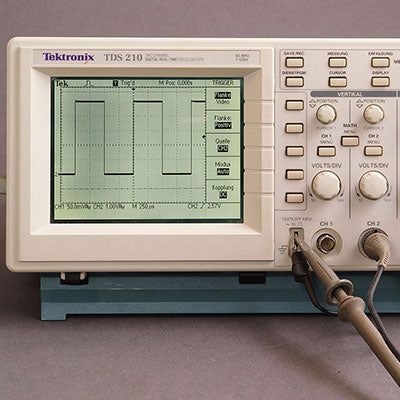#The oscilloscope is a measuring device that allows us to see changes in electrical voltage that depend on time. We know that a voltmeter is used to measure electrical voltage under normal conditions. The voltmeter can only show us the magnitude and direction of the voltage in the circuit numerically. On the other hand, the oscilloscope offers an easier way to understand the change of voltage depending on time as a two-axis graph.

## What Does An Oscilloscope Do?

Oscilloscope in daily life; It provides electronic information to be displayed on the screen in applications such as radar systems, television receivers, and computers.

Parts in a simple oscilloscope:

• Cathode ray tube
• Vertical deflector system
• Time base generator,
• Timing circuit,
• Horizontal deflector system,
• External intensity overlay
• High and low voltage power supplies.Cathode ray tube : The cathode ray tube is the most important unit of the oscilloscope and it provides the formation of the image. The cathode ray tube consists of three main parts:

1. Electron gun and focusing-acceleration elements
2. Vertical and horizontal deflection plates
3. Vacuum housing with a phosphor screen that shines when the electron beam hits

Vertical deflection system :
This system contains an attenuator and several amplifiers. Thus, it is ensured that a very wide input voltage is applied to the oscilloscope and a good image is obtained on the screen by increasing the small voltages. In addition, amplifiers provide both the same value gain and minimum distortion at very wide frequencies. High sensitivity is achieved in small bandwidths. The amplitude of the output signal drops to 70.7% of the input signal amplitude at the band end frequency.

Time base generator :
Oscilloscopes are used to measure voltage variations (signals) over time. This occurs when the luminous spot in the chaotic ray tube scans the screen linearly from left to right at a constant speed. Horizontal deflector side is applied to vertical plates (saw tooth tension is applied), it is also called scanning generator. In this way, the signal moves linearly from left to right in the horizontal direction. In the vertical direction, it deviates upwards or downwards according to the backward applied to the horizontal plates.Synchronization circuit :
If we want to see a pattern on the oscilloscope, one period of the scanning voltage must either be completely equal to the period of the signal we want to examine or be in multiples of it. If the pattern moves from right to left on the oscilloscope screen, it means that the scanning voltage has much more periods than the period of the signal that we want to examine. If the signal we want to examine has a slightly lower period of the period of the signal, then the scan voltage shifts the pattern from left to right.
If we want the pattern to remain constant on the screen, the scanning voltage period must be equal to or exactly the times of the signal we want to examine. This situation is called synchronization.
In some cases, if you want to see more than one period of the signal on the screen, the following should be done: For example, you want to see 5 periods. The period of your scanning voltage should be 5 times the period of the signal you are examining.
In addition, connecting the vertical amplifier to the synchronization circuit, the triggering moment is monitored and a synchronization can be created between the vertical input signal horizontal scanning circuit.

Horizontal deflection system :
They consist of horizontal amplifiers and several attenuators. The function of the amplifiers is to raise the applied signal with minimal distortion. The attenuator is used to reduce the amplitude of the input signal.
3 types of input signals can be applied to the horizontal system. 2 external and 1 internal.

External intensity modultiob or z-axis modulation :
Changing the voltage applied to the grid of the cathode ray tube changes the intensity of the light spot or light trace on the screen. By applying a sufficiently large signal to the grid, a discrete trace occurs on the screen. The reason is that the amplitude of the signal applied to the grid increases in one half period and decreases in the other half period. This effect is called the z-axis overlay and the change of light intensity in this way is called the third bozut or z-axis.### What Oscilloscope Measures?

• AC - DC Voltages
• AC- DC Indirect Currents
• Period and Frequency
• Comparison of Multiple Signals and Phase Difference Measurement in Multichannel Oscilloscopes
• Component Test

### Advantages of Oscilloscope

• Input impedance is high
• It measures voltages in the wide frequency band with the same precision.
• It shows the voltage value regardless of the waveform.
• It shows the maximum value of the voltage to be measured. If the signal is sinusoidal, the maximum value is calculated from the voltmeter. If the signal is not sinusoidal, it cannot be measured.